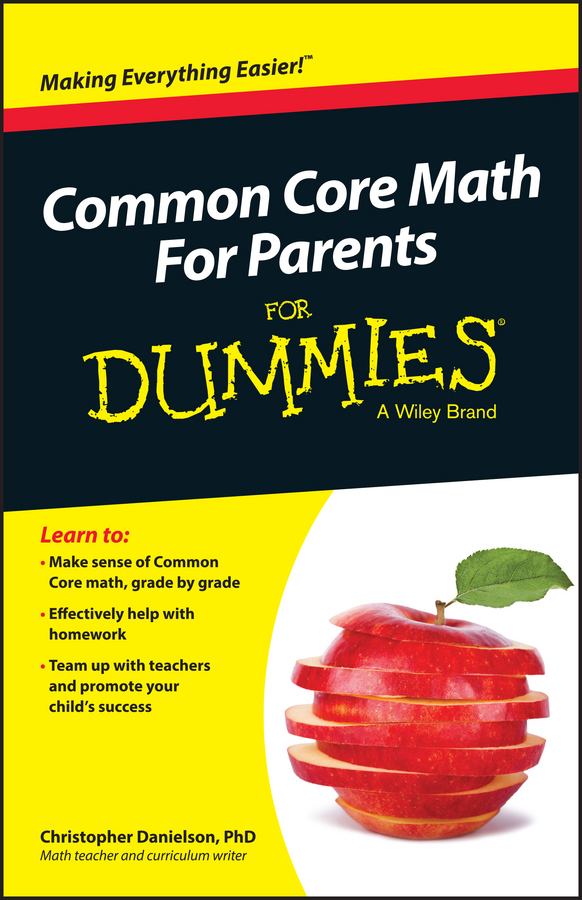##### Common Core Math For Parents For Dummies with Videos OnlineThe least common multiple (LCM) of a set of numbers is the lowest positive number that is a multiple of every number in that set. For example, the LCM of the numbers 2, 3, and 5 is 30 because

• 30 is a multiple of 2 (2 · 15 = 30)

• 30 is a multiple of 3 (3 · 10 = 30)

• 30 is a multiple of 5 (5 · 6 = 30)

• No number lower than 30 is a multiple of all three numbers

Here you will learn two ways to find the LCM of two or more numbers.

## Method 1: Use the multiplication table to find the LCM

To find the LCM of a set of numbers, take each number in the set and jot down a list of the first several multiples in order. The LCM is the first number that appears on every list.

When looking for the LCM of two numbers, start by listing multiples of the higher number, but stop this list when the number of multiples you’ve written down equals the lower number. Then start listing multiples of the lower number until one of them matches the first list.

For example, suppose you want to find the LCM of 4 and 6. Begin by listing multiples of the higher number, which is 6. In this case, list only four of these multiples because the lower number is 4.

Multiples of 6: 6, 12, 18, 24 , . . .

Now, start listing multiples of 4:

Multiples of 4: 4, 8, 12 , . . .

Because 12 is the first number to appear on both lists of multiples, 12 is the LCM of 4 and 6.

This method works especially well when you want to find the LCM of two numbers, but it may take longer if you have more numbers. When working with three numbers, first multiply the two lowest numbers. For the second-highest number, find the product of the other two numbers and list that many multiples. Repeat for the lowest number.

Suppose, for instance, you want to find the LCM of 2, 3, and 5. Again, start with the highest number — in this case, 5 — listing six numbers (the product of the other two numbers, 2 · 3 = 6):

Multiples of 5: 5, 10, 15, 20, 25, 30 , . . .

Next, list multiples of 3, listing ten of them (because 2 · 5 = 10):

Multiples of 3: 3, 6, 9, 12, 15, 18, 21, 24, 27, 30 , . . .

The only numbers repeated on both lists are 15 and 30. In this case, you can save yourself the trouble of making the last list because 30 is obviously a multiple of 2, and 15 isn’t. So 30 is the LCM of 2, 3, and 5.

## Method 2: Use prime factorization to find the LCM

A second method for finding the LCM of a set of numbers is to use the prime factorizations of those numbers. Here’s how:

1. List the prime factors of each number.

Suppose you want to find the LCM of 18 and 24. List the prime factors of each number:

18 = 2 · 3 · 3

24 = 2 · 2 · 2 · 3

2. For each prime number listed, underline the most repeated occurrence of this number in any prime factorization.

The number 2 appears once in the prime factorization of 18 but three times in that of 24, so underline the three 2s:

18 = 2 · 3 · 3

24 = 2 · 2 · 2 · 3

Similarly, the number 3 appears twice in the prime factorization of 18 but only once in that of 24, so underline the two 3s:

18 = 2 · 3 · 3

24 = 2 · 2 · 2 · 3

3. Multiply all the underlined numbers.

Here’s the product:

2 · 2 · 2 · 3 · 3 = 72

So the LCM of 18 and 24 is 72. This checks out because

18 · 4 = 72

24 · 3 = 72